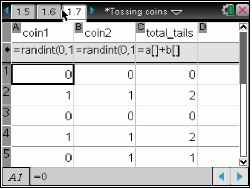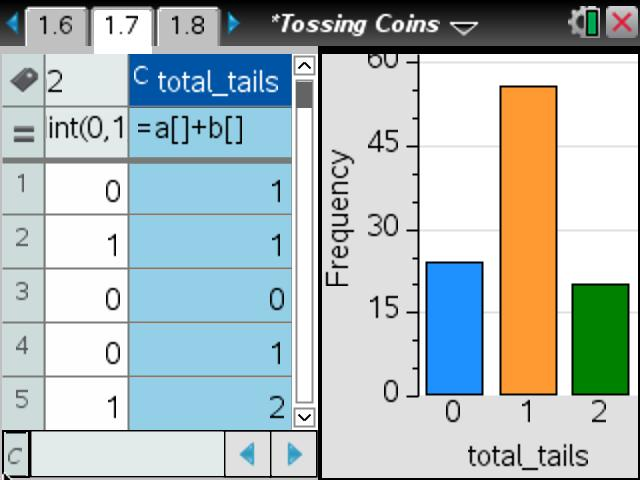# Activities

••• ##### Subject Area

• Math: Statistics: Probability and Random Variables

• ##### Author9-12

45 Minutes

• ##### Device
•TI-Nspire™ CX/CX II
•TI-Nspire™ CX CAS/CX II CAS
• TI-Nspire™ Navigator™
• ##### Software

TI-Nspire™
TI-Nspire™ CAS

3.9

## Tossing Coins

#### Activity Overview

In this activity, students will use a simulation to find the experimental probability of independent events, tossing two coins. They will find the sample space and then compare the experimental and theoretical probabilities.

#### Key Steps

•To start the activity, students are to predict what they think will be the probabilities of the outcomes of tossing a coin twice.

•Then, to quickly determine the sums of 0, 1, and 2 tails, students should use the Quick Graph feature. Students then calculate the experimental probabilities of tossing 0, 1, and 2 tails.